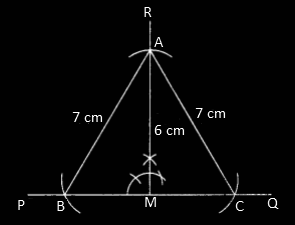# RBSE Maths Class 9 Chapter 8: Important Questions and Solutions

RBSE Maths Chapter 8 -Construction of Triangles Class 9 Important questions and solutions are given here. The important questions and solutions of Chapter 8, provided at BYJU’S, have steps of construction for each and every problem. Also, RBSE Class 9 solutions are available here to help the students get maximum marks in their exams.

RBSE Chapter 8 of the Class 9 Maths has different cases in the construction of triangles with the given parameters. The concepts covered include the construction of a triangle when three sides are given, two sides and one angle is given, two angles and one side is given and so on. Besides, students can also get the steps for that particular construction.

### RBSE Maths Chapter 8: Exercise 8.1 Textbook Important Questions and Solutions

Question 1: Construct a triangle ABC in which AB = 4 cm, BC = 5 cm and CA = 6 cm.

Solution:

Steps of construction: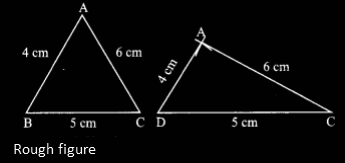(i) Draw a line segment BC = 5 cm.

(ii) With B as the centre, draw an arc of radius 4 cm.

(iii) With C as can be, draw an arc of radius 6 cm which cuts the former arc at A.

(iv) Join AB and AC.

Hence, ∆ABC is the required triangle.

Question 2: Two points A and B are at a distance of 6.5 cm to each other. Find the position of point C which is at a distance of 7 cm and 6 cm from points A and B, respectively.

Solution:

Steps of construction:

(i) Draw base AB = 6.5 cm.

(ii) Take A and B as centre, draw arcs of radii 7 cm and 6 cm above the line and below the line which intersects at C and C’.

(iii) Join AC, BC and C’A and C’B.

Hence, ∆ABC and ∆ABC’ are the required triangles.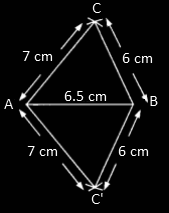Question 3: Construct a triangle ABC in which sides a = 6.5 cm, b = 7.2 cm, c = 8 cm. Draw the bisector of ∠B which meets AC at point M.

Solution:

Steps of construction:

(i) Draw the line segment AC of length 7.2 cm.

(ii) Taking A as the centre, draw an arc of radius 8 cm.

(iii) Again by taking C as the centre, draw an arc of radius 6.5 cm which cuts the previously drawn arc at B.

(iv) Join AB and AC.

(v) Now triangle ABC is obtained.

(vi) Draw BM as the bisector of ∠B which meets AC at M.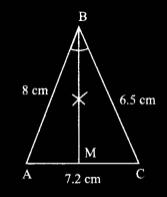Question 4: Construct a ΔABC such that a = 7 cm, b = 5 cm and c = 4 cm. Draw a perpendicular from A on BC.

Solution:

Steps of construction:

(i) Draw the side of the triangle, a = 7 cm.

(ii) Taking B as the centre, draw an arc of radius 4 cm.

(iii) Taking C as the centre C, draw another arc of radius 5 cm which cuts the previous arc at A.

(iv) With centre A, draw perpendicular on BC i.e. AP.

(v) Join AB, AC and AP.

Hence, ∆ABC is the required triangle.Question 5: Construct an equilateral triangle ABC whose each side is 5.5 cm.

Solution:

Steps of construction:

(i) Draw the line segment BC of length 5.5 cm.

(ii) Taking B as the centre, draw an arc of radius of 5.5 cm.

(iii) With C as the centre, draw another arc with the same radius of 5.5 cm which intersects the previously drawn arc at A.

(iv) Join AB and AC.

Hence, ΔABC is the required equilateral triangle.Question 6: Construct an isosceles triangle whose base is 3 cm and equal sides are 5 cm.

Solution:

Steps of construction:

(i) Draw the line segment BC of length 3 cm.

(ii) Taking B as the centre, draw an arc of radius of 5 cm.

(iii) With C as the centre, draw another arc with the same radius which intersects the previously drawn arc at A.

(iv) Join AB and AC.

Hence, ΔABC is the required isosceles triangle.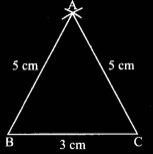### RBSE Maths Chapter 8: Exercise 8.2 Textbook Important Questions and Solutions

Question 7: Construct a triangle ABC in which a = 4 cm, b = 5 cm and ∠C = 60°.

Solution:

Steps of construction:

(i) Draw the line segment AC, i.e. base b = 5 cm.

(ii) Taking C as the centre, draw an angle of 60° with the help of compass.

(iii) With C as the centre, draw an arc of radius 4 cm which cuts the previously drawn angle at A.

(iv) Join AB.

Hence, ΔABC is the required triangle.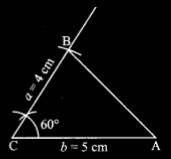Question 8: Construct a triangle LMN in which ∠L =120°, LM = 4 cm, LN = 5 cm.

Solution:

Steps of construction:

(i) Draw the line segment LM = 4 cm.

(ii) Taking L as the centre, draw an angle of 120° with the help of compass.

(iii) With L as the centre, draw an arc of radius 5 cm which cuts the previously drawn angle line at N.

(iv) Join MN.

Hence, ΔLMN is the required triangle.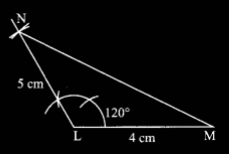Question 9: Construct a triangle ABC in which AB = AC = 8 cm and ∠A = 75°. Also, draw a bisector of ∠B which meets the opposite side.

Solution:

Steps of construction:

(i) Draw the line segment AB = 8 cm as the base.

(ii) Taking A as the centre, draw an angle of 75° with the help of compass.

(iii) With A as the centre, draw an arc of radius 8 cm which cuts the previously drawn angle line at C.

(iv) Join BC.

(v) Draw the angle bisector of B which intersects the opposite side AC at E.

Hence, ΔABC is the required triangle.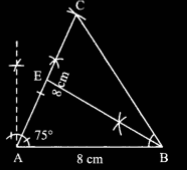Question 10: Construct an isosceles triangle whose vertex angle is 120° and each equal side is of length 5.5 cm.

Solution:

Steps of construction:

(i) Draw one of the equal sides, BC with the length 5.5 cm.

(ii) Taking B as the centre, draw the vertex angle of 120°.

(iii) With B as the centre and radius of 5.5. cm, draw an arc which cuts the angle line at A.

(iv) Join AC.

Hence, ΔABC is the required isosceles triangle.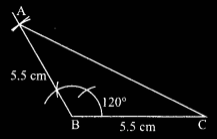### RBSE Maths Chapter 8: Exercise 8.3 Textbook Important Questions and Solutions

Question 11: Construct a triangle PQR in which QR = 8 cm, ∠Q = 120° and ∠R = 30°.

Solution:

Steps of construction:

(i) Draw the line segment QR = 8 cm as the base.

(ii) Taking Q as the centre, draw an angle of 120°.

(iii) With R as the centre, draw an angle of 30° which meets the line of angle 120° at P.

Therefore, ΔPQR is the required triangle.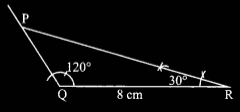Question 12: Construct a triangle ABC in which b = 7 cm, ∠A = 90° and ∠C = 60°.

Solution:

Steps of construction:

(i) Draw the line segment b = 7 cm as the base, i.e. AC.

(ii) Taking A as the centre, draw an angle of 90° using a ruler and compass.

(iii) With C as the centre, draw an angle of 60° which meets the line of angle 90° at B.

Therefore, ΔABC is the required right triangle.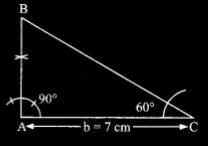Question 13: Construct an isosceles triangle whose base is 4 cm and vertex angle is 30°.

Draw perpendicular from the vertex on base.

Solution:

Given that, vertex angle = 30°

Therefore, each base angle = (180° – 30°)/2 = 150°/2 = 75°

Steps of construction:

(i) Draw a line segment BC of length 4 cm as the base.

(ii) With B as the centre, draw an angle of 75° using a ruler and compass.

(iii) With C as the centre, draw an angle of 75° using ruler and compass which intersects the previously drawn angle line at A.

(iv) Now, draw the perpendicular from vertex A to the base BC.

Hence, ∆ABC is the required isosceles triangle.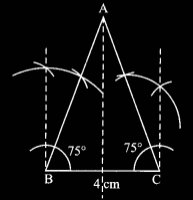### RBSE Maths Chapter 8: Exercise 8.4 Textbook Important Questions and Solutions

Question 14: Construct a right angled triangle whose hypotenuse is 5 cm and the other side is 3 cm.

Solution:

Steps of construction:

(i) Draw a line segment AB of length 3 cm.

(ii) Taking A as the centre, draw an angle line of 90° using a ruler and compass.

(iii) With B as the centre, draw an arc of radius 5 cm which intersects the angle line at C.

(iv) Join BC.

Hence, ∆ABC is the required right triangle.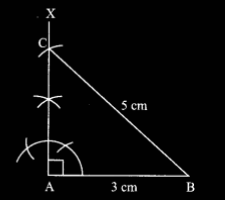Question 15: Construct a triangle ABC in which ∠A = 90°, side AC = 5.4 cm and hypotenuse BC = 10 cm.

Solution:

Steps of construction:

(i) Draw a line segment AC of length 5.4 cm.

(ii) Taking A as the centre, draw an angle line of 90° using a ruler and compass.

(iii) With C as the centre, draw an arc of radius 10 cm which intersects the angle line at B.

(iv) Join BC.

Hence, ∆ABC is the required right triangle.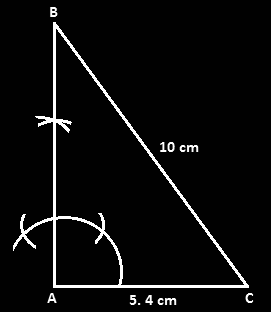Question 16: Construct a right angled triangle ABC in which ∠A = 90° and side a = 10 cm and side b = 6 cm. Draw a perpendicular on hypotenuse from vertex A.

Solution:

Steps of construction:

(i) Draw a line segment AC, i.e. b = 6 cm.

(ii) Taking A as the centre, draw an angle line of 90° using a ruler and compass.

(iii) With B as the centre, draw an arc of radius 10 cm which intersects the angle line at B.

(iv) Join BC.

(v) Again A as the centre, draw the perpendicular bisector of hypotenuse BC.

Hence, ∆ABC is the required right triangle.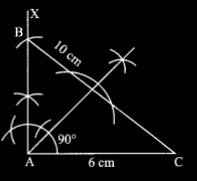### RBSE Maths Chapter 8: Exercise 8.5 Textbook Important Questions and Solutions

Question 17: Construct a ∆XYZ in which ∠XYZ = 60°, XY = 5 cm and XZ = 4.5 cm. How many triangles of such type can be drawn?

Solution:

Steps of construction:

(i) Draw a line YA as the base with some length.

(ii) Taking Y as the centre, draw ∠BYA = 60° using a ruler and compass.

(iii) Taking Y as the centre, draw an arc of radius 5 cm which meets the line BY at X.

(iv) Now, with X as the centre, draw an arc of radius 4.5 cm which intersects the baseline YA at Z.

(iv) Join XZ.

Therefore, the required ∆XYZ is obtained.

Hence, only one triangle is possible with the given parameters.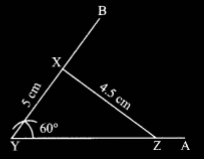Question 18: Construct a triangle PQR in which ∠PQR = 45°, side PQ = 6 cm and PR = 5 cm.

Solution:

Steps of construction:

(i) Draw a line segment PQ of length 6 cm as the base line.

(ii) Taking Q as the centre, draw an angle of 45° using a ruler and compass.

(iii) With P as the centre, draw an arc of radius 5 cm which cuts the angle line at R.

(iv) Join PR.

Hence, ΔPQR is the required triangle.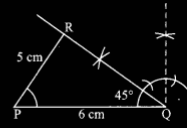Question 19: Construct a triangle ABC in which a = 5.4 cm, b = 6.8 cm and ∠A = 45°. Also, find the measurement of side AB.

Solution:

Steps of construction:

(i) Draw a line AX as the base.

(ii) Draw an angle of 45° at A ( ∠XAY = 45°) using a ruler and compass.

(iii) Taking A as the centre, draw an arc of radius 6.8 cm which intersects AY at C.

(iv) With C as the centre, draw an arc of radius 5.4 cm which cuts the base line AX at B and B’.

(v) Join B and B’ to C.

Hence, ∆AB’C and ∆ABC are the required triangles.

By measuring the length, AB = 7.2 cm (approx)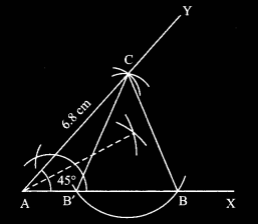### RBSE Maths Chapter 8: Exercise 8.6 Textbook Important Questions and Solutions

Question 20: Construct a triangle ABC in which BC = 7 cm, ∠C = 50° and AC + AB = 8 cm.

Solution:

Steps of construction:

(i) Draw a line segment BC of length 7 cm as the base.

(ii) At the point B, draw an angle of 50°, i.e. ∠BCY = 50° using a ruler and compass.

(iii) Taking C as the centre, draw an arc of length 8 cm which intersects BY at D.

(iv) Join CD.

(v) Draw the perpendicular bisector of BD, intersecting CD at A.

(vi) Join BA.

Hence, ∆ABC is the required triangle.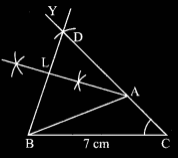Question 21: Construct a triangle PQR in which QR = 5 cm, ∠R = 40° and PR – PQ = 1 cm.

Solution:

Steps of construction:

(i) Draw a line segment QR of length 5 cm.

(ii) Taking R as the centre, construct an angle line RT of 40° and produce it.

(iii) Now, taking R as the centre, draw an arc of radius 1 cm which intersects the angle line RT at S.

(iv) Join QS.

(v) Also, draw the perpendicular bisector of QS which intersects RT at P.

(vi) Join PQ.

Hence, ∆PQR is the required triangle.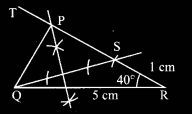Question 22: Construct a triangle ABC whose perimeter is 12 cm and base angles are 50° and 70°.

Solution:

Steps of construction:

(i) Draw a ray PX and cut off a line segment PQ of length 12 cm from it.

(ii) Taking P as the centre, construct ∠YPB = 25° with the help of a protractor (i.e. 1/2 x 50°).

(iii) With Q as the centre, construct ∠ZQP = 35° (i.e. 1/2 x 70°)

(iv) Draw a perpendicular bisector of AP which intersects PQ at B.

(v) Draw a perpendicular bisector of AQ which intersects PQ at C.

(vi) Join AB and AC.

Hence, ∆ABC is the required triangle.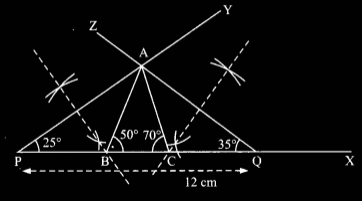Question 23: Construct a triangle ABC in which BC = 6 cm, and medians AD and CF are 6 cm and 7.5 cm, respectively.

Solution:

Steps of construction:

(i) Draw a line segment BC of length 6 cm and mark the midpoint of BC as D.

(ii) AD = 6 cm (given), let G be the centroid then GD = (1/3) x 6 =2 cm. Taking D as the centre, draw an arc of radius 2 cm.

3. CG = (2/3) x CF = (2/3) x 7.5 = 5 cm. By taking C as the centre, cut an arc of radius 5 cm to intersect the previous arc at G.

4. Draw CGF = 7.5 cm.

5. Draw BF and extend the same.

6. With the centre D, draw an arc of radius AD = 6 cm which intersects the line BF (produced) at A.

7. Join AC.

Hence, ∆ABC is the required triangle.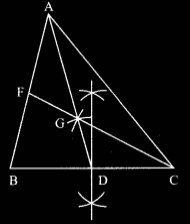### RBSE Maths Chapter 8: Additional Important Questions and Solutions

Question 1: Construct a triangle whose perimeter is 12 cm and ratio of sides is 1 : 2 : 3.

Solution:

Let x, 2x, and 3x be the sides of the triangle.

According to the given,

Perimeter of the triangle = 12 cm

x + 2x + 3x = 12 cm

6x = 12

x = 12/6 = 2

Sides of the triangle are 2 cm, 4 cm, and 6 cm.

Steps of construction:

(i) Draw a line segment BC = 4 cm as the base of the triangle.

(ii) Taking B as the centre, draw an arc of radius 2 cm.

(iii) Taking C as the centre, draw an arc of radius 6 cm, which intersects the previously drawn arc at A.

(iv) Join AB and AC.

Hence, ΔABC is the required triangle.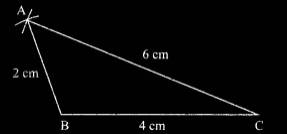Question 2: Construct a triangle ABC in which ∠B = 90°, ∠C = 60° and c = 5 cm.

Solution:

Given,

∠B = 90°, ∠C = 60° and c = 5 cm

That means, AB = 5 cm

∠A = 180° – (90° + 60°) = 180° – 150° = 30°

Steps of construction:

(i) Draw a line segment AB = 5 cm as the base of the triangle.

(ii) Taking B as the centre, construct an angle line of 90° with the help of compass.

(iii) With A as the centre, construct an angle of 30°, which intersects the previous angle line at C.

(iv) Join AC.

Hence, ΔABC is the required triangle.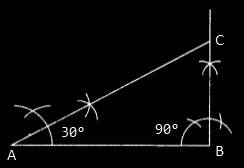Question 3: Construct a right angle triangle ABC in which hypotenuse BC is 8.2 cm and one side is 4.2 cm.

Solution:

Steps of construction:

(i) Draw a line segment AB of length 4.2 cm as the base.

(ii) At point A, draw an angle line of 90°.

(iii) Taking B as the centre, draw an arc of radius 8.2 cm which cuts the previous angle line segment at C.

(iv) Join BC.

Hence, ΔABC is the required triangle.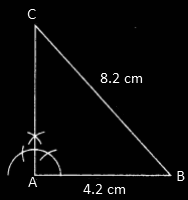Question 4: Construct a triangle ABC in which ∠B = 45°, ∠C = 60° and perpendicular from A on BC is AD and its length is 4 cm.

Solution:

Steps of construction:

(i) Draw a straight line XY as the base line.

(ii) Take point D on the line XY, draw perpendicular DZ.

(iii) By taking D as the centre, cut an arc of radius 4 cm from DZ and marked it as DA.

(iv) Draw ∠DAB = 45° and ∠DAC = 30° which intersect XY at B and C, respectively.

Hence, ΔABC is the required triangle.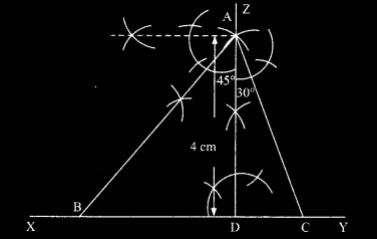Question 5: Construct a triangle ABC in which a = 5.6 cm, b + c = 10.2 cm and ∠B – ∠C = 30°.

[Hint: Vertex angle B is 90°+ (1/2) (∠B – ∠C) = 105°]

Solution:

Steps of construction:

(i) Draw a line segment BC = 5.6 cm as the base.

(ii) Construct an angle line of 105° at B.

(iii) Taking C as the centre, cut an arc of radius 10.2 cm, which intersects the line of ∠CBD at E.

(iv) Join EC.

(v) Draw a perpendicular bisector of EB, which meets CE at A.

(vi) Join AB.

Hence, ΔABC is the required triangle.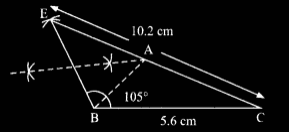Question 6: Construct a triangle whose all three medians are 4.2 m, 4.8 cm and 5.4 cm.

Solution:

Steps of construction:

(i) Draw a line segment AG of length 2.8 cm and produce to a point P such that AG = GP.

(ii) From centre G, cut an arc GC of length 3.6 cm and from P cut an arc of 3.2 cm to intersect each other at C.

GC = (2/3) x CF = (2/3) x 5.4 = 3.6 cm

CP = BG = (2/3) x BE = (2/3) x 4.8 = 3.2 cm

(iii) From the centre G, cut an arc GB = (2/3) x BE = 3.2 cm and from P cut an arc PB = CG = 3.6 cm so that both will intersect each other at B.

(iv) Join BC, AB and AC.

Hence, ΔABC is the required triangle.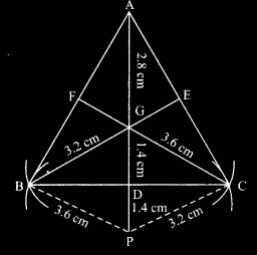Question 7: Construct an isosceles triangle whose height is 6 cm and equal sides are 7 cm. Find the measurement of base.

Solution:

Steps of construction:

(i) Draw a line PQ as the base line.

(ii) Draw a perpendicular on any point say M, on the line PQ.

(iii) Taking M as the centre, draw an arc of radius 6 cm which intersects the perpendicular and mark that point as A.

(iv) From the point A, cut an arc of 7 cm on either side of the perpendicular and mark them as B and C on the base line PQ.

(v) Join AB and AC.

Hence, ΔABC is the required triangle.